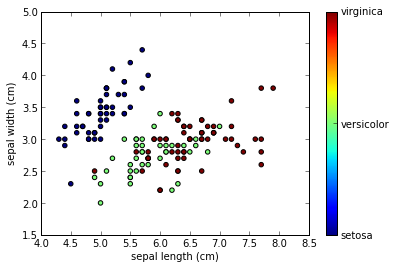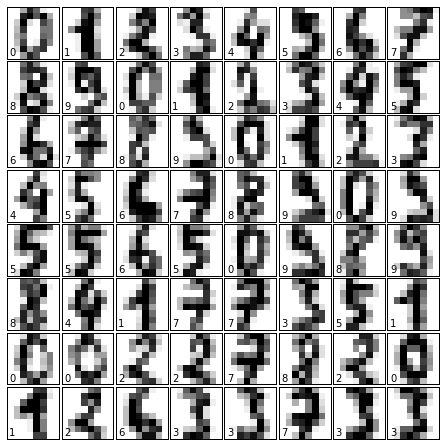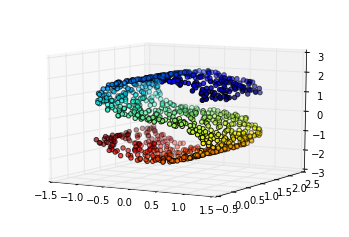# Representation and Visualization of Data¶

Machine learning is about creating models from data: for that reason, we'll start by discussing how data can be represented in order to be understood by the computer. Along with this, we'll build on our matplotlib examples from the previous section and show some examples of how to visualize data.

By the end of this section you should:

• Know the internal data representation of scikit-learn.
• Know how to use scikit-learn's dataset loaders to load example data.
• Know how to turn image & text data into data matrices for learning.
• Know how to use matplotlib to help visualize different types of data.

## Data in scikit-learn¶

Data in scikit-learn, with very few exceptions, is assumed to be stored as a two-dimensional array, of size [n_samples, n_features].

Most machine learning algorithms implemented in scikit-learn expect data to be stored in a two-dimensional array or matrix. The arrays can be either numpy arrays, or in some cases scipy.sparse matrices. The size of the array is expected to be [n_samples, n_features]

• n_samples: The number of samples: each sample is an item to process (e.g. classify). A sample can be a document, a picture, a sound, a video, an astronomical object, a row in database or CSV file, or whatever you can describe with a fixed set of quantitative traits.
• n_features: The number of features or distinct traits that can be used to describe each item in a quantitative manner. Features are generally real-valued, but may be boolean or discrete-valued in some cases.

The number of features must be fixed in advance. However it can be very high dimensional (e.g. millions of features) with most of them being zeros for a given sample. This is a case where scipy.sparse matrices can be useful, in that they are much more memory-efficient than numpy arrays.

### A Simple Example: the Iris Dataset¶

As an example of a simple dataset, we're going to take a look at the iris data stored by scikit-learn. The data consists of measurements of three different species of irises. There are three species of iris in the dataset, which we can picture here:

In :
from IPython.core.display import Image, display
display(Image(filename='figures/iris_setosa.jpg'))
print "Iris Setosa\n"

display(Image(filename='figures/iris_versicolor.jpg'))
print "Iris Versicolor\n"

display(Image(filename='figures/iris_virginica.jpg'))
print "Iris Virginica"Iris SetosaIris VersicolorIris Virginica


### Quick Question:¶

If we want to design an algorithm to recognize iris species, what might the data be?

Remember: we need a 2D array of size [n_samples x n_features].

• What would the n_samples refer to?

• What might the n_features refer to?

Remember that there must be a fixed number of features for each sample, and feature number i must be a similar kind of quantity for each sample.

Scikit-learn has a very straightforward set of data on these iris species. The data consist of the following:

• Features in the Iris dataset:

1. sepal length in cm
2. sepal width in cm
3. petal length in cm
4. petal width in cm
• Target classes to predict:

1. Iris Setosa
2. Iris Versicolour
3. Iris Virginica

scikit-learn embeds a copy of the iris CSV file along with a helper function to load it into numpy arrays:

In :
from sklearn.datasets import load_iris


The resulting dataset is a Bunch object: you can see what's available using the method keys():

In :
iris.keys()

Out:
['target_names', 'data', 'target', 'DESCR', 'feature_names']

The features of each sample flower are stored in the data attribute of the dataset:

In :
n_samples, n_features = iris.data.shape
print n_samples
print n_features
print iris.data

150
4
[ 5.1  3.5  1.4  0.2]


The information about the class of each sample is stored in the target attribute of the dataset:

In :
print iris.data.shape
print iris.target.shape

(150, 4)
(150,)

In :
print iris.target

[0 0 0 0 0 0 0 0 0 0 0 0 0 0 0 0 0 0 0 0 0 0 0 0 0 0 0 0 0 0 0 0 0 0 0 0 0
0 0 0 0 0 0 0 0 0 0 0 0 0 1 1 1 1 1 1 1 1 1 1 1 1 1 1 1 1 1 1 1 1 1 1 1 1
1 1 1 1 1 1 1 1 1 1 1 1 1 1 1 1 1 1 1 1 1 1 1 1 1 1 2 2 2 2 2 2 2 2 2 2 2
2 2 2 2 2 2 2 2 2 2 2 2 2 2 2 2 2 2 2 2 2 2 2 2 2 2 2 2 2 2 2 2 2 2 2 2 2
2 2]


The names of the classes are stored in the last attribute, namely target_names:

In :
print iris.target_names

['setosa' 'versicolor' 'virginica']


This data is four dimensional, but we can visualize two of the dimensions at a time using a simple scatter-plot. Again, we'll start by enabling pylab inline mode:

In :
# note: this also imports numpy as np, imports matplotlib.pyplot as plt, and others
%pylab inline

Welcome to pylab, a matplotlib-based Python environment [backend: module://IPython.zmq.pylab.backend_inline].

In :
x_index = 0
y_index = 1

# this formatter will label the colorbar with the correct target names
formatter = plt.FuncFormatter(lambda i, *args: iris.target_names[int(i)])

plt.scatter(iris.data[:, x_index], iris.data[:, y_index], c=iris.target)
plt.colorbar(ticks=[0, 1, 2], format=formatter)
plt.xlabel(iris.feature_names[x_index])
plt.ylabel(iris.feature_names[y_index])

Out:
<matplotlib.text.Text at 0x109c1b390>### Quick Exercise:¶

Change x_index and y_index in the above script and find a combination of two parameters which maximally separate the three classes.

This exercise is a preview of dimensionality reduction, which we'll see later.

## Other Available Data¶

Scikit-learn makes available a host of datasets for testing learning algorithms. They come in three flavors:

• Packaged Data: these small datasets are packaged with the scikit-learn installation, and can be downloaded using the tools in sklearn.datasets.load_*
• Downloadable Data: these larger datasets are available for download, and scikit-learn includes tools which streamline this process. These tools can be found in sklearn.datasets.fetch_*
• Generated Data: there are several datasets which are generated from models based on a random seed. These are available in the sklearn.datasets.make_*

You can explore the available dataset loaders, fetchers, and generators using IPython's tab-completion functionality. After importing the datasets submodule from sklearn, type

datasets.load_<TAB>



or

datasets.fetch_<TAB>



or

datasets.make_<TAB>



to see a list of available functions.

In :
from sklearn import datasets

In :



The data downloaded using the fetch_ scripts are stored locally, within a subdirectory of your home directory. You can use the following to determine where it is:

In :
from sklearn.datasets import get_data_home
get_data_home()

Out:
'/Users/ogrisel/scikit_learn_data'
In :
!ls \$HOME/scikit_learn_data/

20news-bydate.pkz    cal_housing.pkz      lfw_home             mldata               olivetti.pkz         species_coverage.pkz


Be warned: many of these datasets are quite large, and can take a long time to download! (especially on Conference wifi).

If you start a download within the IPython notebook and you want to kill it, you can use ipython's "kernel interrupt" feature, available in the menu or using the shortcut Ctrl-m i.

You can press Ctrl-m h for a list of all ipython keyboard shortcuts.

Now we'll take a look at another dataset, one where we have to put a bit more thought into how to represent the data. We can explore the data in a similar manner as above:

In :
from sklearn.datasets import load_digits

In :
digits.keys()

Out:
['images', 'data', 'target_names', 'DESCR', 'target']
In :
n_samples, n_features = digits.data.shape
print (n_samples, n_features)

(1797, 64)

In :
print digits.data
print digits.target

[  0.   0.   5.  13.   9.   1.   0.   0.   0.   0.  13.  15.  10.  15.   5.
0.   0.   3.  15.   2.   0.  11.   8.   0.   0.   4.  12.   0.   0.   8.
8.   0.   0.   5.   8.   0.   0.   9.   8.   0.   0.   4.  11.   0.   1.
12.   7.   0.   0.   2.  14.   5.  10.  12.   0.   0.   0.   0.   6.  13.
10.   0.   0.   0.]
[0 1 2 ..., 8 9 8]


The target here is just the digit represented by the data. The data is an array of length 64... but what does this data mean?

There's a clue in the fact that we have two versions of the data array: data and images. Let's take a look at them:

In :
print digits.data.shape
print digits.images.shape

(1797, 64)
(1797, 8, 8)


We can see that they're related by a simple reshaping:

In :
print np.all(digits.images.reshape((1797, 64)) == digits.data)

True


Aside... numpy and memory efficiency:

You might wonder whether duplicating the data is a problem. In this case, the memory overhead is very small. Even though the arrays are different shapes, they point to the same memory block, which we can see by doing a bit of digging into the guts of numpy:

In :
print digits.data.__array_interface__['data']
print digits.images.__array_interface__['data']

(4434440192, False)
(4434440192, False)


The long integer here is a memory address: the fact that the two are the same tells us that the two arrays are looking at the same data.

Let's visualize the data. It's little bit more involved than the simple scatter-plot we used above, but we can do it rather quickly.

In :
# set up the figure
fig = plt.figure(figsize=(6, 6))  # figure size in inches
fig.subplots_adjust(left=0, right=1, bottom=0, top=1, hspace=0.05, wspace=0.05)

# plot the digits: each image is 8x8 pixels
for i in range(64):
ax = fig.add_subplot(8, 8, i + 1, xticks=[], yticks=[])
ax.imshow(digits.images[i], cmap=plt.cm.binary, interpolation='nearest')

# label the image with the target value
ax.text(0, 7, str(digits.target[i]))We see now what the features mean. Each feature is a real-valued quantity representing the darkness of a pixel in an 8x8 image of a hand-written digit.

Even though each sample has data that is inherently two-dimensional, the data matrix flattens this 2D data into a single vector, which can be contained in one row of the data matrix.

## Generated Data: the S-Curve¶

One dataset often used as an example of a simple nonlinear dataset is the S-cure:

In :
from sklearn.datasets import make_s_curve
data, colors = make_s_curve(n_samples=1000)
print(data.shape)
print(colors.shape)

(1000, 3)
(1000,)

In :
from mpl_toolkits.mplot3d import Axes3D
ax = plt.axes(projection='3d')
ax.scatter(data[:, 0], data[:, 1], data[:, 2], c=colors)
ax.view_init(10, -60)

/usr/local/lib/python2.7/site-packages/mpl_toolkits/__init__.py:2: UserWarning: Module argparse was already imported from /usr/local/Cellar/python/2.7.5/Frameworks/Python.framework/Versions/2.7/lib/python2.7/argparse.pyc, but /usr/local/lib/python2.7/site-packages is being added to sys.path
__import__('pkg_resources').declare_namespace(__name__)This example is typically used with an unsupervised learning method called Locally Linear Embedding. We'll explore unsupervised learning in detail later in the tutorial.

## Exercise: working with the faces dataset¶

Here we'll take a moment for you to explore the datasets yourself. Later on we'll be using the Olivetti faces dataset. Take a moment to fetch the data (about 1.4MB), and visualize the faces. You can copy the code used to visualize the digits above, and modify it for this data.

In :
from sklearn.datasets import fetch_olivetti_faces

In :
# fetch the faces data

In :
# Use a script like above to plot the faces image data.
# hint: plt.cm.bone is a good colormap for this data


### Solution:¶

In :
%load solutions/02A_faces_plot.py